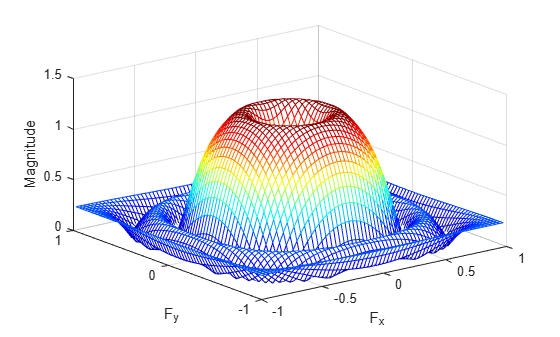# ftrans2

2-D FIR filter using frequency transformation

## Syntax

``h = ftrans2(b,t)``
``h = ftrans2(b)``

## Description

````h = ftrans2(b,t)` produces the two-dimensional FIR filter `h` that corresponds to the one-dimensional FIR filter `b` using the transform `t`. The transform matrix `t` contains coefficients that define the frequency transformation to use.```

example

````h = ftrans2(b)` uses the McClellan transform matrix `t`.t = [1 2 1; 2 -4 2; 1 2 1]/8;```

## Examples

collapse all

Use `ftrans2` to design an approximately circularly symmetric two-dimensional bandpass filter with passband between 0.1 and 0.6 (normalized frequency, where 1.0 corresponds to half the sampling frequency, or π radians). Since `ftrans2` transforms a one-dimensional FIR filter to create a two-dimensional filter, first design a one-dimensional FIR bandpass filter using the Signal Processing Toolbox function `firpm`.

```colormap(jet(64)) b = firpm(10,[0 0.05 0.15 0.55 0.65 1],[0 0 1 1 0 0]); [H,w] = freqz(b,1,128,'whole'); plot(w/pi-1,fftshift(abs(H)))```Use `ftrans2` with the default McClellan transformation to create the desired approximately circularly symmetric filter.

```h = ftrans2(b); freqz2(h)```## Input Arguments

collapse all

FIR filter, specified as a numeric matrix. `b` must be a 1-D Type I (even symmetric, odd-length) filter such as can be returned by `fir1` (Signal Processing Toolbox), `fir2` (Signal Processing Toolbox), or `firpm` (Signal Processing Toolbox).

Data Types: `double`

The transform matrix, specified as a numeric matrix. `t` contains coefficients that define the frequency transformation to use. By default, `ftrans2` uses a McClellan transform matrix.

Data Types: `double`

## Output Arguments

collapse all

2-D FIR filter, returned as a numeric matrix. `ftrans2` returns `h` as a computational molecule, which is the appropriate form to use with `filter2`. If `t` is `m`-by-`n` and `b` has length `Q`, then `h` is size `((m-1)*(Q-1)/2+1)`-by-`((n-1)*(Q-1)/2+1)`.

## Algorithms

The transformation below defines the frequency response of the two-dimensional filter returned by `ftrans2`.

`${{H}_{\left({\omega }_{1},{\omega }_{2}\right)}=B\left(\omega \right)|}_{\mathrm{cos}\omega =T\left({\omega }_{1},{\omega }_{2}\right)},$`

where B(ω) is the Fourier transform of the one-dimensional filter `b`:

`$B\left(\omega \right)=\sum _{n=-N}^{N}b\left(n\right){e}^{-j\omega n}$`

and T(ω1,ω2) is the Fourier transform of the transformation matrix `t`:

`$T\left({\omega }_{1},{\omega }_{2}\right)=\sum _{{n}_{2}}\sum _{{n}_{1}}t\left({n}_{1},{n}_{2}\right){e}^{-j{\omega }_{1}{n}_{1}}{e}^{-j{\omega }_{2}{n}_{2}}.$`

The returned filter `h` is the inverse Fourier transform of H(ω1,ω2):

`$h\left({n}_{1},{n}_{2}\right)=\frac{1}{{\left(2\pi \right)}^{2}}{\int }_{-\pi }^{\pi }{\int }_{-\pi }^{\pi }H\left({\omega }_{1},{\omega }_{2}\right){e}^{j{\omega }_{1}{n}_{1}}{e}^{j{\omega }_{2}{n}_{2}}d{\omega }_{1}d{\omega }_{2}.$`

 Lim, Jae S., Two-Dimensional Signal and Image Processing, Englewood Cliffs, NJ, Prentice Hall, 1990, pp. 218-237.